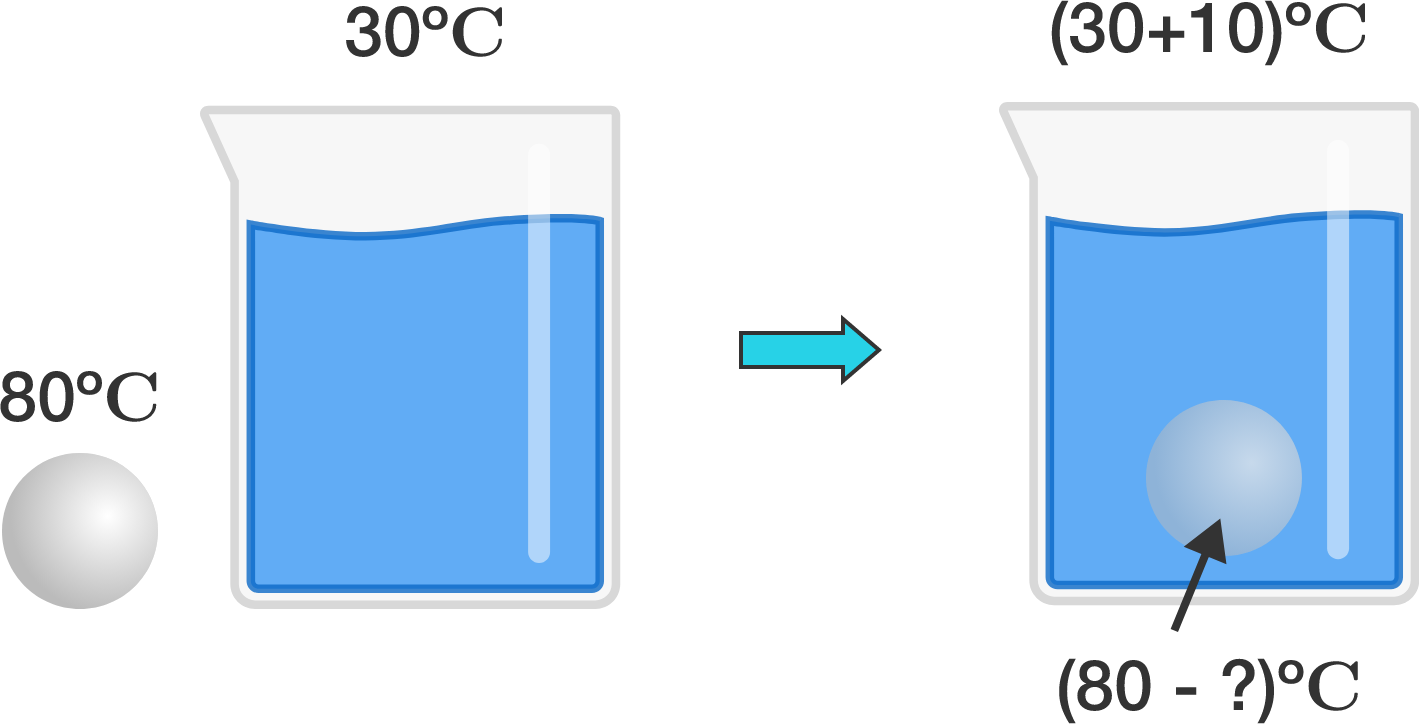# Hot ball in cold waterA metal ball with temperature $80 ^\circ\text{C}$ is dropped in water with temperature $30 ^\circ\text{C}$. The metal ball and water exchange heat with each other, and after a while both their temperatures become constant.

Assuming that no heat has been lost to or gained from the surroundings and the container, if the water temperature has been raised by $10 ^\circ\text{C}$, what is the decrease in the temperature of the ball?

×